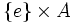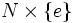# Disproving intersection-closedness

This survey article is about proof techniques for or related to: subgroup metaproperty satisfaction
Find other survey articles about subgroup metaproperty satisfaction

This article lists some common techniques, ideas, and underlying themes, behind proofs that certain subgroup properties are not intersection-closed. While some of these techniques involve a direct construction of counterexamples, others simply use the fact that the subgroup does not satisfy various corollaries of being intersection-closed.

For strategies to prove the opposite (i.e., that a subgroup property is intersection-closed), refer proving intersection-closedness.

## The various version of intersection-closed

• Intersection-closed subgroup property: This is a subgroup property that is closed under arbitrary nonempty intersections. In other words,$p$ is intersection-closed if the intersection of a nonempty collection of subgroups of$G$, each satisfying property$p$ in$G$, also satisfies property$p$ in$G$. For a complete list, see Category:Intersection-closed subgroup properties.
• Strongly intersection-closed subgroup property: An intersection-closed subgroup property that is also identity-true, i.e., it is satisfied by any group as a subgroup of itself. In particular, this property is closed under intersection of arbitrary collections, including possibly the empty collection. (The intersection of an empty collection of subgroups is the whole group).
• Finite-intersection-closed subgroup property: A subgroup property that is closed under taking intersections of finite nonempty collections.
• Strongly finite-intersection-closed subgroup property: A subgroup property that is finite-intersection-closed and identity-true.

## The direct product technique

### Outline of the technique

This method is used to try to construct an example of an intersection of direct factors which does not satisfy a given property$p$. It thus could work for properties that are implied by the property of being a direct factor but are not implied by the property of normality.

(If it is implied by normality, then any intersection of direct factors would have the property).

The idea is to take the direct product of$G$ with an abelian group$A$, such that we have in mind a surjective homomorphism$\rho: G \to A$ with kernel a subgroup$N$ of$G$.

Now we consider the automorphism$\sigma:G \times A \to G \times A$ defined as$(g,a) \mapsto (g,\rho(g)a)$. Note that this automorphism fixes$\{ e \} \times A$ pointwise.

Consider the subgroup$(G \times \{ e \}) \cap \sigma(G \times \{ e \})$. This is precisely$N \times \{ e \}$ where$N$ is the kernel of$\rho$. Thus, it suffices to show that$N \times \{ e \}$ does not satisfy the property$p$ in$G \times A$.

### How this technique is applied

Suppose$p$ is a property such that:

• Any direct factor satisfies property$p$
•$p$ satisfies the intermediate subgroup condition
• There is a group$G$ with a normal subgroup$N$ that does not satisfy$p$, and such that the quotient$G/N$ is abelian.

Then, the above technique constructs for us an intersection of direct factors which does not satisfy the property$p$. More precisely, let$A$ be an abelian group isomorphic to$G/N$. Construct a homomorphism$\rho:G \to A$ with kernel$N$, and obtain that$N \times \{ e \}$ is an intersection of direct factors of$G \times A$. Since every direct factor satisfies$p$,$N \times \{ e \}$ satisfies$p$ in$G \times A$. Since$p$ satisfies the intermediate subgroup condition,$N \times \{ e \}$ satisfies$p$ in the intermediate subgroup$G \times \{ e \}$, so$N$ satisfies$p$ in$G$. This contradicts the assumption.

Another way of saying this is that if a subgroup property is satisfied by direct factors, satisfies the intermediate subgroup condition, and is intersection-closed, then it must be satisfied by every Abelian-quotient subgroup.

Examples of properties to which this technique can be applied:

### The technique shows more

The two direct factors that we intersect are in fact automorphs, because the map$\sigma$ is an automorphism of$G \times A$. Thus, the technique shows that the above properties are not finite-automorph-intersection-closed.

## The core technique

### The basic result

If a subgroup property$p$ is intersection-closed, then the normal core of any subgroup with property$p$ also has property$p$. So does the characteristic core.

Thus, in particular, any intersection-closed subgroup property is normal core-closed as well as characteristic core-closed.

### Application to NCI-subgroup properties

A subgroup property is termed a NCI-subgroup property if the only normal subgroup that satisfies it is the whole group. Clearly, if a subgroup property is NCI, then the only way it can be normal core-closed is if it is satisfied by the whole group only.

Thus, to show that a subgroup property is not normal core-closed, it suffices to show that it is a NCI-subgroup property and we can find an example of a group and a proper subgroup satisfying the property in it.

Examples of properties for which this approach works:

## Using left residuals

This is a somewhat more sophisticated technique.

### The basic idea: left residuals preserve behavior under intersections

Suppose$p$ and$q$ are two subgroup properties. The left residual of$p$ by$q$ is the subgroup property$r$ defined as follows. A subgroup$H$ of a group$K$ has property$r$ in$K$ if for any group$G$ containing$K$ as a subgroup with property$q$,$H$ has property$p$ in$G$.

The following turn out to be true:

• Intersection-closedness is left residual-preserved: If$p$ is intersection-closed, so is the left residual of$p$ by any property$q$.
• Finite-intersection-closedness is left residual-preserved: If$p$ is closed under finite intersections, so is the left residual of$p$ by any property$q$.

Thus, to prove that a property$p$ is not closed under intersections (finite or arbitrary), it suffices to prove that the left residual of$p$ by some property$q$ is not closed under intersections (finite or arbitrary).